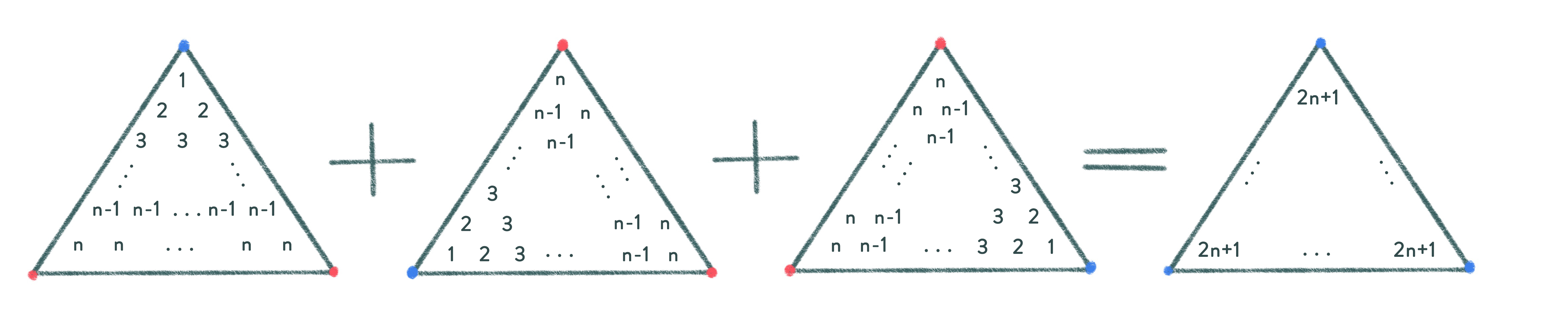## Solutions

Problem 1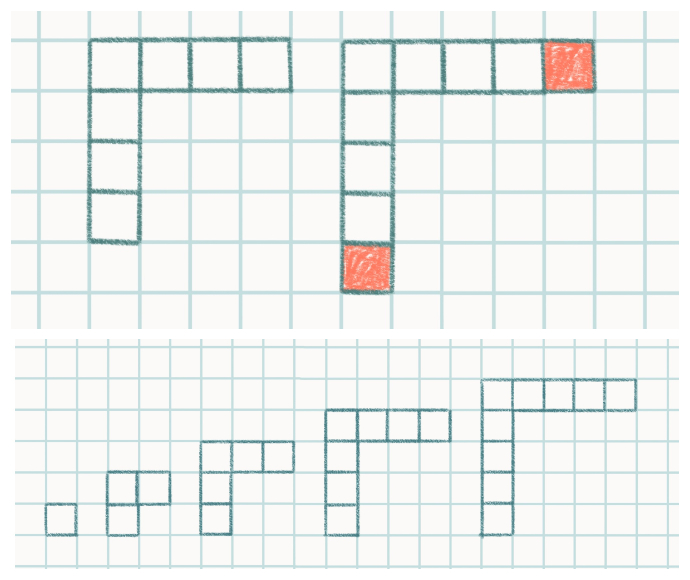This diagram shows corners. Let’s imagine n such corners. Each new corner is two squares bigger than the one preceding it (see picture on the right). In the picture below it, the first corner is made up of one square only, the second corner is 3 squares, the third corner is 5 etc. The number of squares in each new corner is also the next odd number. The first n corners make up a square n x n. Therefore, the corners altogether contain n2 squares. As a result, the sum of the first n odd numbers equals n2.

Note. The nth corner contains n + (n - 1) = 2n – 1 squares (see diagram 6). For this reason, the nth odd number equals 2n - 1, and we get the following equation 1 + 3 + 5 + ... + (2n - 1) = n2

Problem 2

Solution: There are 8 more.

Problem 3

If there are 8 squares inside the blue frame (this we can count), then there are 8 + 8 = 2 × 8 squares inside the green frame, 3 × 8 squares inside the orange one, 4 × 8 squares inside the purple one etc. Now, we can gather the squares from the central frame.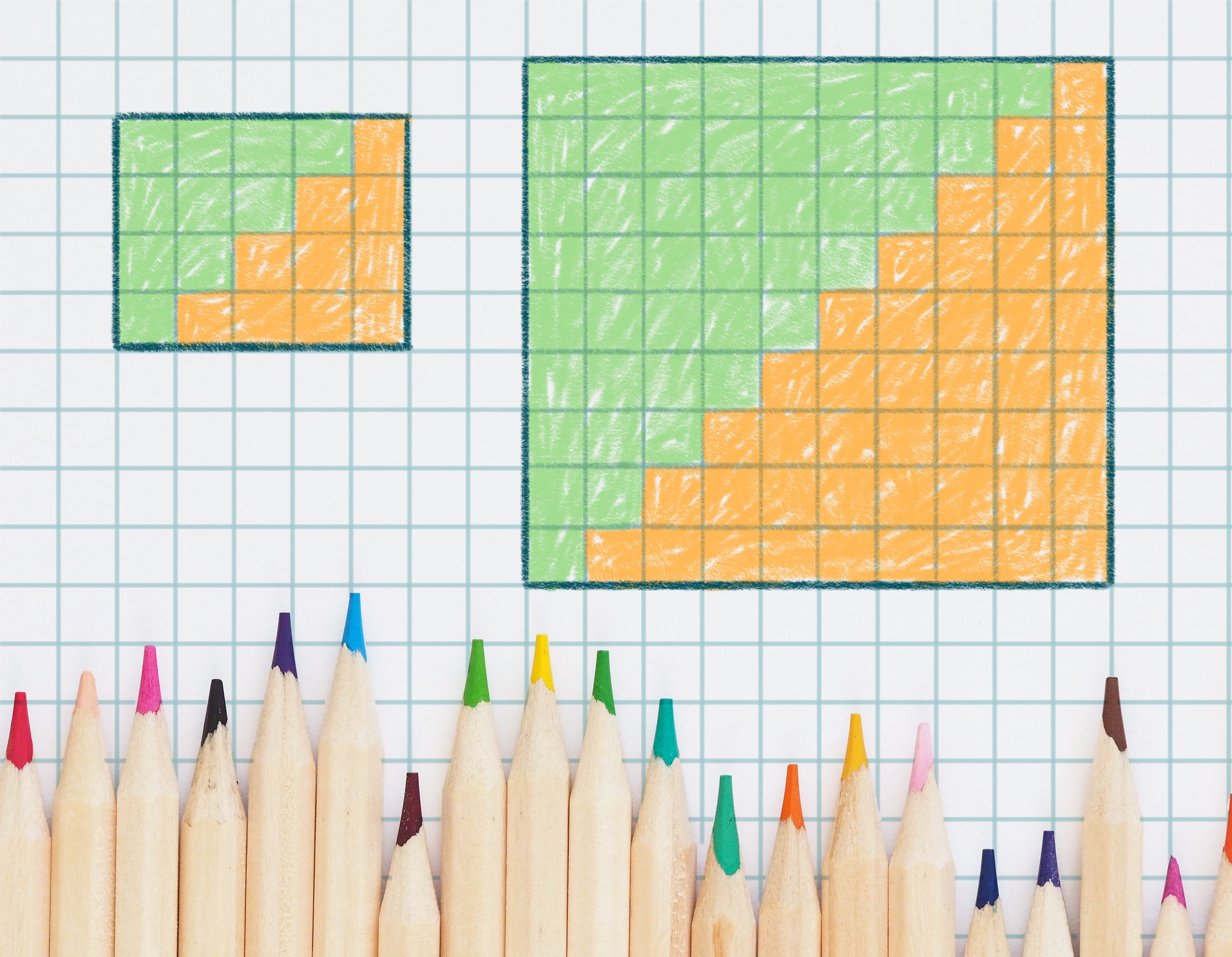Problem 4

a) See diagram on the right.

b) It shows how two identical shapes -- the little staircases of any dimensions – can come together to make a rectangle. In our mind, let’s do this with two such staircases containing n steps. In this case, we get a rectangle with dimensions (n + 1) × n.

It follows that each little staircase contains ((n + 1) × n) / 2 squares. On the other hand, when one counts the squares in a staircase in rows, then there are (1 + 2 + ... + n) squares. Therefore, (1 + 2 + ... + n) = ((n + 1) n) / 2.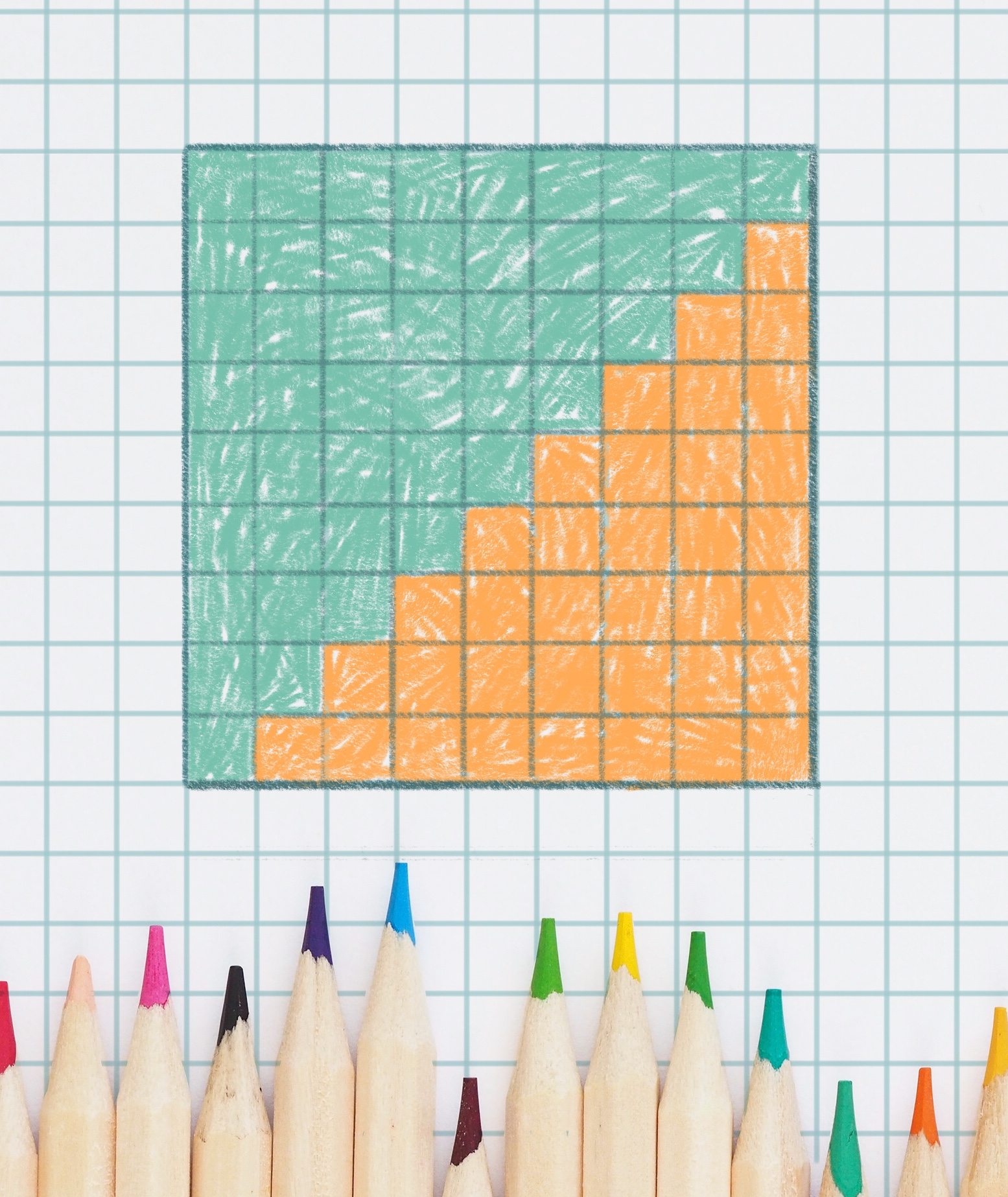Problem 5

See diagram on the right.

Problem 6

a) In this case, the kth corner is broken up into many rectangles with one side of each being equal to k. (For example, the green corner is fourth, and it is divided into rectangles with one side equal to 4). The other sides of these rectangles equal (moving top-down and then from right to left) 1, 2, … (k - 1), k, (k - 1), (k - 2), … 2, 1. We know (see problem 7) that the sum of these numbers 1 + 2+ ... + (k - 1) + k + (k - 1) + ... + 2 + 1 equals k2. For this reason, if we are to divide this corner into the rectangles described above, then we can use these rectangles to create one with the sides k2 and k. This means that the corner k contains k3 squares altogether.

b) The multiplication table of Pythagoras is divided into ‘thick’ corners containing 13, 23 ... n3 squares (or cells). Therefore, we need to count the number of cells in the table. The table is a square, with a side equal to 1 + 2 + ... + n. This sum (already calculated in problem 6) is equal to                     (n × (n + 1)) / 2.

It follows that 13 + 23 + … + n3 = (n2 × (n + 1)2) / 4.

If the problems above have not managed to tire you out, please enjoy the ideas below as a bonus:

Problem 7.

a) When counting the number of squares of each color in this diagram, you get the following equation:

12 + 22 + … + n2 = 1 × n + 3 × (n - 1) + 5 × (n - 2) + 7 × (n - 3) + … + (2n - 1) × 1

b) Now, add diagram 15 to the diagram 14 to find 12 + 22 + … + n2.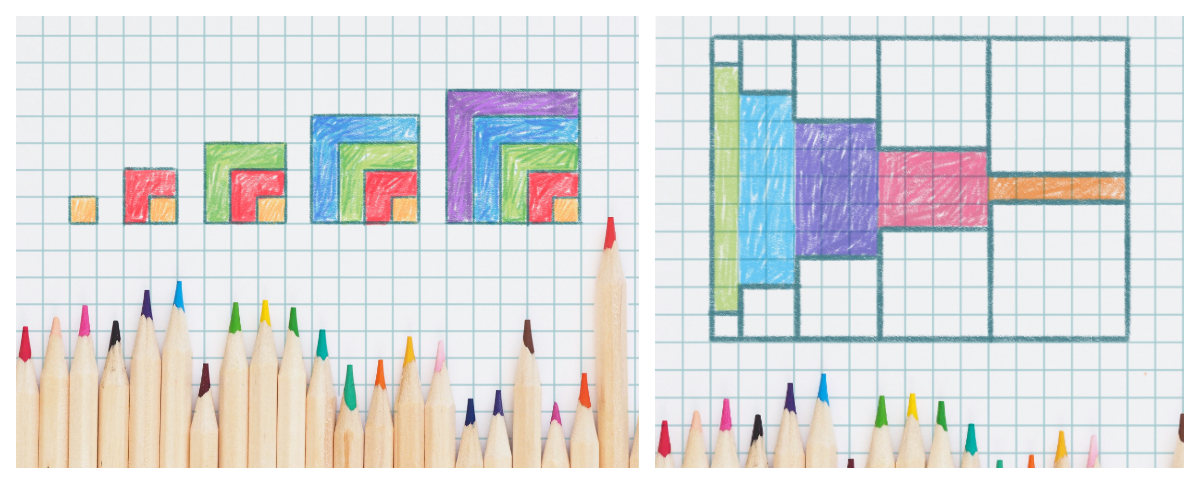Problem 8.

Explain the equality shown in diagram 16, and you will get another method for finding the equation describing the sum of squares 12 + 22 + … + n2.